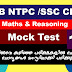A2Z Academy offers a wide range of online mock test series for many SSC, RRB and Kerala PSC examinations. Practicing A2Z Academy’s Previous Year Mock Test series will induce confidence in the students to attempt the actual exam. Level up your preparation for RRB NTPC & SSC CHSL   exams by attempting below free online mock tests. We suggest you to try answering the questions yourself. Daily Mock test available here in various subject like Current Affairs, General Knowledge, General English, Quantitative Aptitude and Reasoning.

(RRB NTPC & SSC CHSL Maths ) Mock Test -19

01. At the rate of 12% for 1 and ½ year on Rs.1,25,000. how much compound interest will be charged if the interest is compounded half yearly?
24000
23877
22500
20000

02․ What will be the compound interest on Rs.1,25,000 at the rate of 8% per annum of 9 months if compound interest to be calculated quarterly.
7500
7651
8300
7750

03․ In an examination 70% of the candidates passed in English. 80% passed in Maths. 10% failed in both the subjects. If 144 candidates passed in both, the total number of candidates were:
240
250
300
600

04․ In an examination, a student must get 36% marks to pass. A student who gets 190 marks failed by 35 marks. The total marks in that examination is
600
650
625
700

05․ Two candidates contested in an election. One got 60% of the votes and won by 1600 votes. What is the number of votes polled?
7500
8000
10000
6000

06․ In a examination A got 25% marks more than B, B got 10% less than C and C got 25% more than D. If D got 320 marks out of 500, the marks obtained by A were
360
450
400
500

07․ In an election between two candidates, the candidate getting 60% of the votes polled, is elected by a majority of 14,000 votes. The number of votes polled by the winning candidates
42000
32000
30000
50000

08․ In an office 40% of the staff is female, 40% of the female and 60% of the male voted for me. The percentage of votes I got was
80
60
52
55

09․ A man took a loan from a bank at the rate of 12% per annum at simple interest. After 3 years he had to pay Rs.5,400 as interest only for the period. The principal amount borrowed by him was:
18000
20000
15000
13000

10․ A person deposited Rs.400 for 2 years, Rs.550 for 4 years. He received the total simple interest of Rs.1020. The rate of interest per annum is.
30%
20%
33%
34%

11․ Rs.500 was invested at 12% per annum simple interest and a certain sum of money invested at 10% per annum simple interest. If the sum of the interest on both the sum after 4 years is Rs.480, the latter sum of money is:
400
450
600
500

12․ A lent Rs.5000 to B for 2 years and Rs.3000 to C for 4 years on simple interest at the same rate of interest and received Rs.2200 in all from both as interest. The rate of interest per annum is:
15
10
12
20

13․ A lends Rs.2500 to B and a certain sum to C at the same time at 7% annual simple interest. If after 4 years, A altogether receives Rs.1120 as interest from B and C, the sum lent to C is
2000
3000
1500
2500

14․ The difference between the selling prices of an article sold at 4% and 3% profits is Rs.3. The cost price of the articles is:
400
300
250
500

15․ A man sells two articles at Rs.99 each. On one he gains 10% and on the other he loses 10%. What is his gain or loss percent on the whole transaction?
1% Profit
1%loss
10%Loss
10%Profit

16․ A man gains 20% by selling an article for a certain price. If he sells it at double the price, the percentage of profit will be:
130%
140%
40%
20%

17․ A dealer makes a profit of 20% even after giving a 10% discount on the advertised price of a scooter. If he makes a profit of Rs.7500 on the sale of the scooter, the advertised price was
25000
35000
50000
55000

18․ A sells an article to B at a gain of 10%, B sells it to C at a gain of 5%. If C pays Rs.462 for it, what did it cost of A?
450
400
350
300

19․ A and B can d a work in 12 days, B and C in 15 days and C and A in 20 days. If A,B and C work together, the y will complete the work in:
60
60
10
20

20․ A and B together can complete a work in 8 days and B and C together in 12days. All of the three together can complete the work in 6 days. In how much time will A and C together complete the work?
12
10
8
15

Railway NTPC & SSC CHSL Maths Mock Test - 19Reviewed by Admin on Tuesday, March 12, 2019 Rating: 5